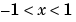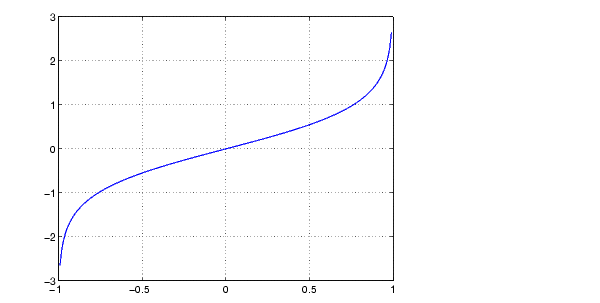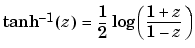MATLAB Function Referenceatanh

Inverse hyperbolic tangent

Syntax

• ```Y = atanh(X)
```

Description

The `atanh` function operates element-wise on arrays. The function's domains and ranges include complex values. All angles are in radians.

```Y = atanh(X) ``` returns the inverse hyperbolic tangent for each element of `X`.

Examples

Graph the inverse hyperbolic tangent function over the domain.

• ```x = -0.99:0.01:0.99;
plot(x,atanh(x)), grid on
```Definition

The hyperbolic inverse tangent can be defined as

•Algorithm

`atanh` uses FDLIBM, which was developed at SunSoft, a Sun Microsystems, Inc. business, by Kwok C. Ng, and others. For information about FDLIBM, see http://www.netlib.org.

See Also

`atan2`, `atan`, `tanh`

© 1994-2005 The MathWorks, Inc.